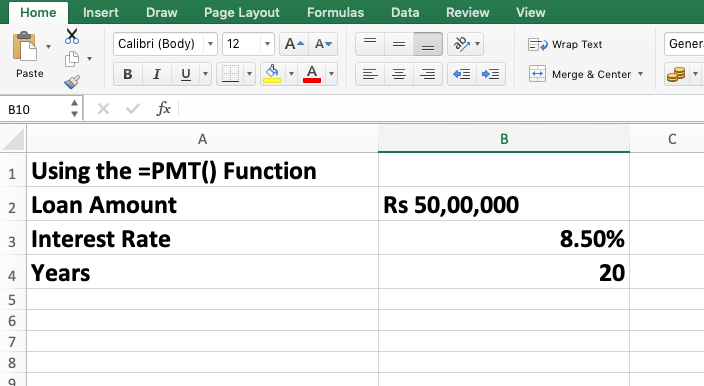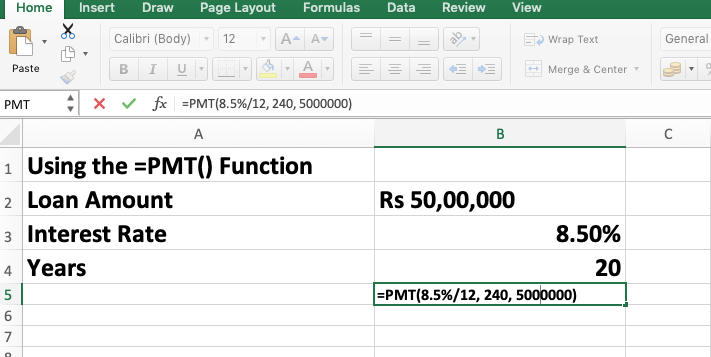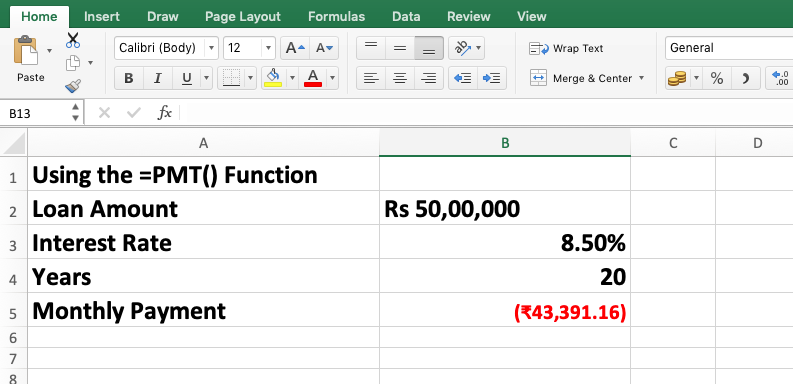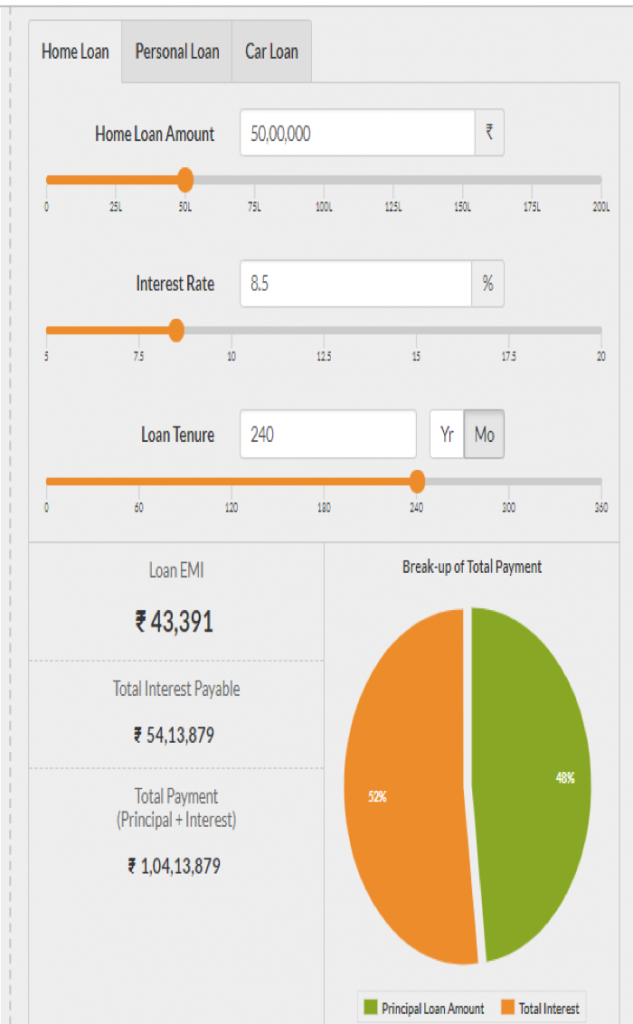📲
How EMI Is Calculated

# How EMI Is Calculated(Shutterstock)

Easy availability of home loans has made it possible for a large part of India’s population to realise its dream of owning a house. However, you must take due care while using this opportunity for your advantage. It is in this context that in this article we discuss what EMI is and how it is calculated.

Explaining EMI

Banks grant home loans depending on your repayment capacity, which they gauge through a number of checks. Depending on that, financial institutions fix a tenure, through the course of which you have to repay your loan – the principal, along with interest. The payments are typically made monthly through equated monthly installments, or EMIs.

Of course, your bank calculates the EMI and lets you know the monthly payment you are liable to make. However, it would be better if you know how to calculate home loan EMI and do some match beforehand on your own – for two reasons. First, this will help you understand what kind of additional expense you will be incurring every month after your EMIs start. Also note here that ideally, only 40 per cent of your in-hand salary should be spent towards home loan EMI payment.

And second, you will be able to verify the calculation done by your bank and ask for corrections, if necessary. Without further ado, let us now get to the task of making the calculations.

How is the home loan EMI calculated?

There are three ways to do that.

First, you could make the calculation using a simple mathematical formula.

Second, you could do it using an excel sheet.

And third, you could use online EMI calculators to do the task for you.

Mathematical formula and its application: The basic formula used to arrive at your monthly EMI figure is…

EMI = P x R x [{(1 + R)^N} / {1 – (1+R)^N}]

Here,

• P stands for principal loan
• R stands for your monthly interest rate [(annual rate/12)/100]
• N stands the total number of months during the loan tenure

Now, let us understand the calculation through an example.

Suppose Ram took a home loan of Rs 50 lakh at an 8.50 per cent interest rate for a 20-year loan tenure. What would be his EMI? He can calculate it like this:

R = [(annual rate /12)/100]

= (8.5/12)/100

= 0.70/100

= 0.0070

N = 240

EMI = P x R x [{(1 + R)^N}/{1 – (1+R)^N}]

= 50,00,000 x 0.00708333 x [{(1 + 0.00708333)^240}/{1-(1 + 0.00708333^240)}]

= 50,00,000 x 0.00708333 x [{5.44123824}/{4.44123824}]

= 50,00,000 x 0.00708333 x [1.22516243]

EMI = Rs 43,391.16

The PMT formula:  The present value of payments (PMT) function in Excel allows you to use the feature to calculate your EMI. The formula to do so is, PMT (r, nper, pv).

• R here stands for rate of interest (8.50 per cent)
• NPER stands for the number of months over which the money has to be paid. (240 months)
• PV stands for the present value of the loan, meaning the principal amount (Rs 50,00,000)

Here is a step-by-step process that would help you reach the calculation:

In the sheet, the first key in your loan amount, interest rate and number of years. Note that while keying in the loan amount, you have to mention the currency, and while mentioning the interest rate, you have to key in the percent sign. Here you could mention the tenure in year terms.Below this, key in the formula PMT= r, nper, pv). Note that here you have to key in the loan tenure on a monthly basis.Press enter and you get the EMI that you need to pay.Using online EMI calculators: Besides almost all banks, several financial service providers also offer free online tools for EMI calculation. This is possibly the easiest way to calculate the home loan EMI. All you have to do is to key in the loan amount, interest rate and loan tenure. These tools also tell you the exact loan break-up for you.Source: emicalculator.net

Last Updated: Wed Dec 05 2018

## Similar articles

@@Wed May 13 2020 19:59:51